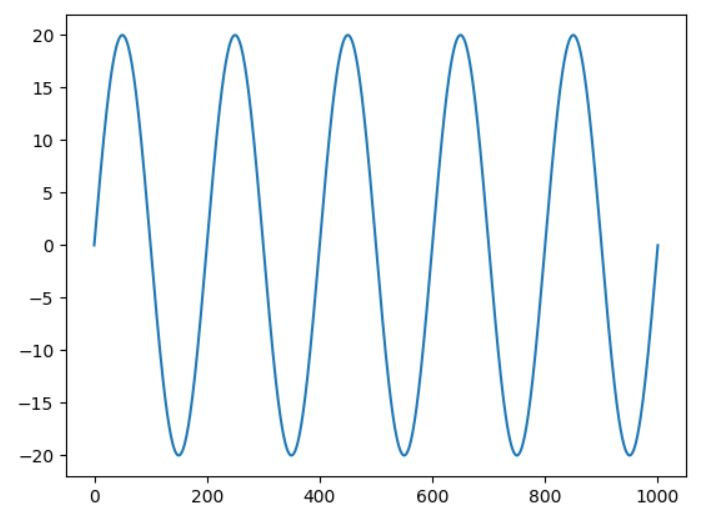# matplotlib

plot[plɑt] : 陰謀

matplotlib是Python著名的繪圖庫, 包含了類似matlab一整套的繪圖api. pylab則為matplotlib裏的一個子項目. 所以要使用pylab的話, 需先安裝matplotlib

import pylab as plt
plt.legend()
plt.show()

plt.legend()其實一點也不傳奇, 因為只要使用 plt.plot(x, y) 把二個list的值傳進去, plt.show()就會顯示出來. 但如果連x, y的值都沒有, 那就用 legend()來代替

# numpy

numpy是常用的套件. 在Windows下直接下達 pip install numpy即可, 但在ubuntu下, pip安裝有bug無法使用, 請使用如下指令安裝

sudo pip3 uninstall numpy
sudo apt install python3-numpy

# numpy.linspace

linspace 全文為 linear space, 線性分割的意思

numpy.linspace(x, y, n) : 在x 及y 之間, 產生n-2 個數. 也就是說, 包含頭尾的 x 及 y, 再加上中間的數字, 總共n 個. 每個數都是等差級數. 所以總共有 (n-1) 個間隔, 每個間隔的大小(公差)為 (y-x)/(n-1)

numpy.linspace(1,9,5)

numpy.linspace(0,1,10)

[0. 0.11111111 0.22222222 0.33333333 0.44444444 0.55555556
0.66666667 0.77777778 0.88888889 1. ]

# numpy.random.random(n)

a=numpy.random.random(10)
print (a)

[0.0120728 0.53486766 0.94797098 0.64184738 0.57318404 0.32338013
0.41668946 0.9873259 0.73820014 0.33671621]
a=np.random.random(10)*10
print (a)

# 繪製直線

import pylab as plt
import numpy as np
x=np.linspace(0,10,10)
value=x*3/10+5#一階方程式, 斜率為 3/10
plt.figure(figsize=(6,6))#設定圖形大小
plt.xlim(-5,15)#設定x軸的範圍
plt.ylim(0,20)#設定y軸的範圍
plt.plot(x,value)
plt.show()

# 繪製圓型

import pylab as plt
import numpy as np
n=360
angle=np.linspace(0,n,n) #切成360等份
x_axis=n*np.cos(np.pi*angle/180) #x座標
y_axis=n*np.sin(np.pi*angle/180) #y座標
plt.figure(figsize=(6,6)) #設定長寬大小
plt.plot(x_axis, y_axis)
plt.show()

# 正弦波

import pylab as plt
import numpy as np
n=1000
x_axis=np.linspace(0,n,n)
y_axis=20*np.sin(10*np.pi*x_axis)
plt.plot(x_axis, y_axis)
plt.show()